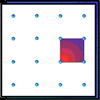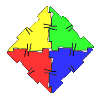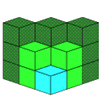#### You may also like### Geoboards

This practical challenge invites you to investigate the different squares you can make on a square geoboard or pegboard.### Polydron

This activity investigates how you might make squares and pentominoes from Polydron.If you had 36 cubes, what different cuboids could you make?

# Halloween Investigation

##### Age 7 to 11Challenge Level

Ali worked hard at this problem and sent us a spreadsheet of the solution.  Ali says:

The way we solved the problem is as follows:
1) First, we put all the data into a table.
2) We worked out the combinations for each possibility, then we started to see patterns forming in our solutions table.  For example, we saw that in the "masks" column, there is an alternating pattern of "green" and "Dracula".
3) Then, we recognised the same logic in the "Hats" column and the "Cloak" column.  There is no variation in the "Gown" column since there is only one type of gown.
4) The answer to the first question is $24$ combinations.

If we look at Ali's spreadsheet, we can see that there are $24$ combinations using:
a black cloak or a wizard's cloak
the grey gown
six different hats (what Ali has called pointy, wizard, crown, Santa, school and muslim)

5) We used the same method for the next question (using the two new masks, what Ali has called 'old' and 'clown'), but in the spreadsheet we used red to distinguish this part of the question from the others.
6) The answer to the second question is another $24$ combinations, making the total of $48$ combinations.

7) We used the same method for the third question, using yellow to denote the new information on the yellow cloak, as well as the combinations found using this new information.
8) The answer to the third question is another $24$ combinations, making the total of $72$ combinations.

9) For the last part (with addition of the shoes), we added the new information in green in our table.
10) Then we worked through the combinations as before, noticing the repeating patterns.
11) The answer to the fourth question is a grand total of $360$ combinations.

Wow - you have worked very systematically, Ali - well done!